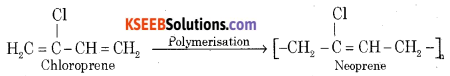# 2nd PUC Chemistry Previous Year Question Paper March 2020

Students can Download 2nd PUC Chemistry Previous Year Question Paper March 2020, Karnataka 2nd PUC Chemistry Model Question Papers with Answers helps you to revise the complete Karnataka State Board Syllabus and score more marks in your examinations.

## Karnataka 2nd PUC Chemistry Previous Year Question Paper March 2020

Time: 3:15 Hours
Max. Marks: 70

Instructions

• The question paper has four parts A, B, C, and D. All parts are compulsory.
• Write balanced chemical equations and draw labeled diagrams wherever required.
• Use Jog tables and simple calculators if necessary (Use of scientific calculators is not allowed).

Part – A

I. Answer all the following: (10 × 1 = 10)

Question 1.
What is the value of Van’t Hoff factor (i) for K2SO4?
$$\mathrm{K}_{2} \mathrm{SO}_{4} \rightarrow 2 \mathrm{K}^{+}+\mathrm{SO}_{4}^{2-}$$
i = $$\frac{3}{1}$$ = 3

Question 2.
10 mL of liquid A is mixed with 10 mL of liquid B, the volume of the resultant solution is 19.9 mL. What type of deviation is expected from Raoult’s law?
Negative or -ve

Question 3.
What is a secondary cell?
A secondary cell after use can be recharged so that it can be used again.Question 4.
Identify the order of the reaction from the rate constant K = 2.3 × 10-6 L mol-1 s-1.
Second-order reaction

Question 5.
Give reason. Zeolites are the good shape-selective catalysts.
ZSM – 5

Question 6.
Iron scraps are economically cheaper than Zinc Scraps.

Question 7.
Complete the reaction
XeF6 + H2O → ____ + 2HF
XeOF4

Question 8.
Give reason. In the case of optically active alkyl halides, SN1 reactions are accompanied by racemization.
Due to the inversion of configuration.Question 9.
Identify ‘A’ in the reaction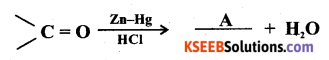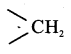Question 10.
Give an example of a water-soluble vitamin.
Vitamin – C.

Part – B

II. Answer any FIVE questions: (Each question carries two marks)

Question 11.
Calculate the no. of particles present per unit cell in a B.C.C unit cell.
Number of particles (atoms) in BCC unit cell = $$\frac {1}{8}$$ × 8 (at corners) + 1 (at corners)
= 1 + 1
= 2 particles

Question 12.
A solution of Ni(NO3)2 is electrolyzed between platinum electrodes using a current of 5 amperes for 20 minutes. What mass of nickel is deposited at the cathode? [molar mass of Ni = 58.7 gram mol-1].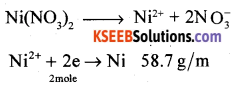Question 13.
Mention any two factors which influence the rate of the reaction.

• The concentration of the reactants or pressure of the reactants
• Temperature
• Catalyst
• Nature of the reactants and their physical states.

Question 14.
Give two reasons. The chemistry of actinoids is more complicated than Lanthanoids.

• are prepared in nanograms.
• have very less half-lives.Question 15.
How is phenol prepared from Aniline? Write the equation.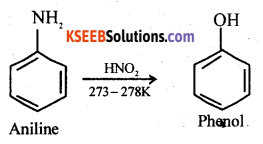Question 16.
Explain Cannizzaro’s reaction taking benzaldehyde as an example.
Aldehyde which does not have an α-hydrogen atom, undergo self-oxidation and reduction reaction on heating with conc. alkali. In this reaction, one molecule of the aldehyde is reduced to alcohol while another is oxidized to carboxylic acid salt.Question 17.
(a) Give an example of nonnarcotic analgesics.
Analgesics are chemicals used to reduce body pain without causing impairment of consciousness.
Examples:

• Non-narcotic analgesic – Aspirin
• Narcotic analgesic – Morphine.

(b) Why the use of Aspartame is limited to cold foods and soft drinks?
Unstable to heat hence limited to cold foods and soft drinks.

Question 18.
(a) Why detergents with a straight chain of hydrocarbons are preferred over branched-chain hydrocarbons?
Straight chain hydrocarbons are more soluble in water, easily forms micelle so are more easily decomposed than those, branched-chain hydrocarbons are one.(b) Give one example for detergent with straight-chain hydrocarbon.
Sodium dodecylbenzene – sulphonate
Anionic detergents are sodium salts of sulfonated long-chain alcohols or hydrocarbons.
Ex:
(i) Sodium lauryl sulphate
CH3(CH2)10 CH2OSO3Na
(ii) Sodium dodecylbenzene sulphonateThese are used in toothpaste.

Part – C

Answer any FIVE of the following: (Each question carries three marks) (5 × 3 = 15)

Question 19.
Write the equations involved in the leaching of alumina from bauxite ore.
(a) Bauxite ore is heated with a concentrated solution of NaOH at 473K to 523K and 35-36 bar pressure. Al2O3 dissolves out as sodium aluminates leaving behind impurities.
Al2O3 + 2NaOH + 3H2O → 2Na[AI(OH)4]
(b) The aluminate in solution is neutralized by passing CO2 gas and hydrated Al2O3 is precipitated.
2Na[Al(OH)4] + 2CO2 → Al2O3 . 3H2O + 2NaHCO3
(c) Hydrated alumina is filtered, dried, and heated to give pure alumina.
Al2O3 . 3H2O $$\underrightarrow { { Al }_{ 2 }{ O }_{ 3 }(1770K) }$$ Al2O3 + 3H2O

Question 20.
Mention any three anomalous properties of nitrogen.
Nitrogen shows the following anomalous properties.

• The smaller size
• High electronegativity.
• High ionization enthalpy.
• Non-availability of d-orbitals.Question 21.
In the manufacture of sulphuric acid write
(i) The equation with the condition for the oxidation of SO2 to SO3.
(ii) Formation of Oleum from SO3.
(i) Catalytic oxidation of SO2 with O2 in presence of V2O5 catalyst gives SO3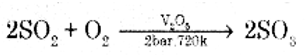(ii) SO3 gas is absorbed in Conc. H2SO4 to produce oleum. Dilution of oleum with water gives H2SO4 of the desired concentration.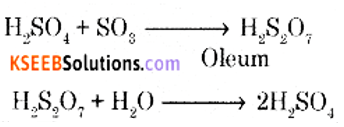Question 22.
(a) Complete the following reaction.
(i) NH3 + 3Cl2 → ______ + 3HCl
Ammonia reacts with excess of chlorine forming nitrogen trichioride.
NH3 + 3Cl2 → NCl3 + 3HCl

(ii) Cl2 + F2 $$\underrightarrow { 473K }$$ ______
2ClF

(b) Write the structure of perchloric acid (HClO4).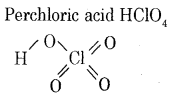Question 23.
(a) Transition elements show the catalytic property. Give two reasons.
Transition metal and its compounds are known for their catalytic activity.
This is because of transition metals.

• Form intermediate complexes.
• Lowers the activation energy of the reaction.(b) Name one 3d series element that does not show a variable oxidation state.
(Zn)/Zinc.

Question 24.
Write the equation for the manufacture of potassium dichromate from chromite ore.
Step – 1: Potassium dichromate is prepared by fusion of chromite ore [FeOCr2O3] with sodium carbonate with an excess of air.
4FeO.Cr2O3 + 8Na2CO3 + 7O2 → 2Fe2O3 + 8Na2CrO4 + 8CO2

Step – 2: The yellow solution of sodium chromate is filtered and acidified with sulphuric acid. The orange solution of sodium dichromate is obtained.
2Na2CrO4 + 2H+ → NaCr2O7 + H2O + 2Na+

Step – 3: The sodium dichromate solution is treated with a potassium chloride solution to get potassium dichromate.
Na2Cr2O7 + 2KCl → K2Cr2O7 + 2NaCl
Orange crystals of potassium dichromate crystalize out.

Question 25.
Using valence bond theory explain geometry hybridization and magnetic property of [CoF6]3-
[Give atomic no. of Co: 27]
(i) Orbitals of Co3+ ion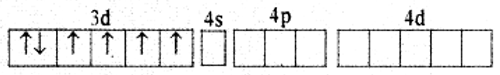(ii) In Co3+ ion sp3d2 hybridization takes place d2 hybridized orbitals of Co3+(iii) Six pairs of electrons, one from each F-ion occupy the six hybrid orbitals. Thus, the complex has octahedral geometry and paramagnetic in nature due to the presence of four unpaired electrons.For [CoF6]3- Complex:
(i) Type of hybndisation : sp3d2
(ii) Geometry : Octahedral
(iii) Magnetic property : Paramagnetic.

Question 26.
(a) Mention any two postulated of Werner’s theory of coordination compounds.

1. In co-ordination compounds, metal atom shows two types of valencies.
• Primary valency
• Secondary valency.
2. The primary valency is ionizable and is satisfied by negative ions.
3. The secondary valency is non-ionizable and is satisfied by negative ions or neutral molecules.
4. The primary valency is the oxidation number and secondary valency is the co-ordination number of the metal atom.(b) Indicate the type of Isomerism in the following set of complex compounds.
[CO(NH3)5SCN]Cl2 and [CO(NH3)5NCS]Cl2.

Part – D

IV. Answer any three of the following: (Each question carries five marks) (3 × 5 = 15)

Question 27.
(a) Calculate the packing efficiency in F.C.C. cubic lattice.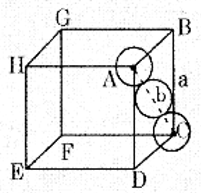Let ‘a’ : be the edge length of the cube
‘b’ : be the face diagonal
‘r’ : is the radius of the sphere b = 4r
In ∆ABC
b2 = a2 + a2
b2 = 2a2
b = √2 × a
4r = √2 × a
a = $$\frac{4}{\sqrt{2}}$$ r
a = 2√2 r
Volume of the cube = a3 = (2√2 r)3
Each unit cell of CCP structure contain 4 spheres.
Volume of the four spheres = 4 × $$\frac{4}{3} \pi r^{3}$$Packing Efficiency = 74%

(b) Calcium metal crystallizes in a face-centered cubic lattice with an edge length of 0.556nm. Calculate the density of the metal. [Atomic mass of calcium 40 g/mol. NA = 6.022 × 1023 atoms/mol].Question 28.
(a) The Vapour pressure of benzene is 200 mm of Hg. When 2 grams of a non-volatile solute dissolved in 78-gram benzene. Benzene has a vapour pressure of 195 mm of Hg. Calculate the molar mass of the solute. [Molar mass of benzene is 78 gram mol-1].
8 gm/mol

(b) What are azeotropes? Give an example for binary solutions showing minimum boiling azeotrope.
Binary mixture boils at the same temperature without undergoing any change in composition is known as an azeotropic mixture.
Example: Mixture of ethanol and water.

The solution at a specific composition has a constant boiling point Less than either of the two pure components is called minimum boiling azeotrope.
Example: 95% ethanol and 5% water forms minimum boiling azeotrope at a constant temperature of 78°C.Question 29.
(a) Calculate the e.m.f. of the cell in which the following reaction takes place.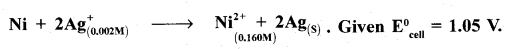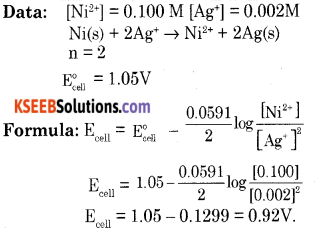(b) (i) State Kohlrausch’s law of Independent Migration of ions.
Limiting the molar conductivity of an electrolyte can be represented as the sum of the individual contributions of the anion and cation of the electrolyte.
If the molar conductivity of cation is denoted by $$\lambda_{+}^{0}$$ and that of anion $$\lambda_{-}^{0}$$
$$\wedge_{m}^{\circ}=v_{+} \lambda_{+}^{0}+v_{-} \lambda_{-}^{0}$$
Where v+ and v are the number of cations and anions per formula unit of an electrolyte.

(ii) What is meant by limiting molar conductivity?
Molar conductivity of an electrolyte solution at infinite dilution (when concentration approaches zero) is called limiting molar conductivity.

Question 30.
(a) Derive an integrated rate equation for a first-order reaction.
Consider a zero-order reaction.
R → P
Rate = $$\frac{-d[R]}{d t}$$ = k[R]0
where k is rate constant for zero order reaction.
$$\frac{-d[R]}{d t}$$ = k
d[R] = -k . dt
Integrating both sides
∫d[R] = -∫k . dt
[R] = -kt + 1 ……. (1)
I is constant in integration.
At t = 0, [R] = [R]0 where [R]0 is initial concentration of the reactant.
Substituting in equation (1)
[R]0 = -k × 0 + 1; I = [R]0
Substituting ‘I’ value in equation (1)
[R] = -kt + [R]0
k = $$\frac{[\mathrm{R}]_{0}-[\mathrm{R}]}{t}$$

(b) According to collission theory write two factors responsible for effective collisions.

• The activation energy of the reactant.
• Proper orientation of the molecules.Question 31.
(a) Write a note on Dialysis.
It is a process of removing a dissolved substance from a colloidal solution by means of diffusion through a suitable membrane.The bag of suitable membrane containing the colloidal solution is suspended in a vessel through which freshwater is continuously flowing. The molecules and ions diffuse through the membrane into the outer water and the pure colloidal solution is left behind.

(b) What is the effect on ∆H and ∆S during the process of adsorption?
During adsorption ∆H becomes -ve [decreases], ∆S becomes +ve [increases] and ∆G becomes -ve [decreases].

(c) Give an example of heterogeneous catalysis.
A catalytic process in which the reluctant and the catalyst are in different phases is known as heterogeneous catalysis.
Example (1): Oxidation of sulphur dioxide to sulphur trioxide in presence of platinum catalyst.Example (2): Combination of hydrogen and nitrogen to form ammonia.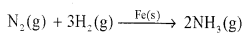Example (3): Hydrogenation of vegetable oil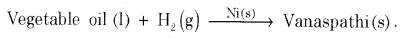V. Answer any four of the following. Each question carries five marks: (4 × 5 = 20)

Question 32.
(a) Explain the SN-1 mechanism for the conversion of tertiary butyl bromide to tertiary butyl alcohol.
The reaction between tert-butyl bromide and hydroxide ion gives tert-butyl alcohol. The rate of reaction depends only on the concentration of tert-butyl bromide. Hence it is a nucleophilic first order substitution reaction.Mechanism:
Step 1: Polarised C – Br undergoes cleavage to produce planar carbocation.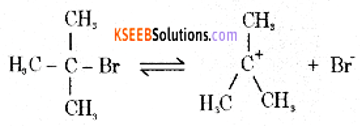Step 2: The carbocation is then attacked by a nucleophile (OH) on either side to form tort-butyl alcohol.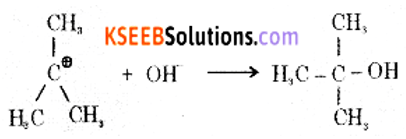(b) Complete the following reactions: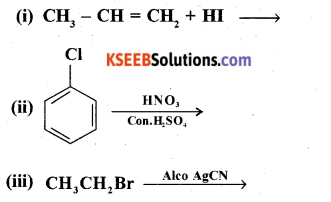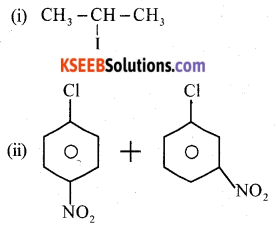(iii) CH3CH2Br + AgCN → CH3CH2NC + AgBrQuestion 33.
(a) Explain the mechanism of acid-catalyzed dehydration of ethanol to ethene.
Step 1: Formation of protonated alcohol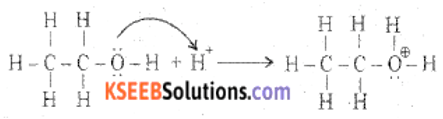Step 2: Formation of carbocation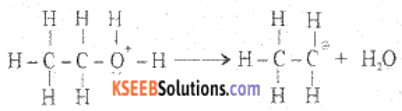Step 3: Formation of ethene by the elimination of a proton(b) How does anisole react with methyl chloride?
Anisole undergoes bromination with bromine in ethanoic acid to form ortho bromoanisole and para-bromoanisole.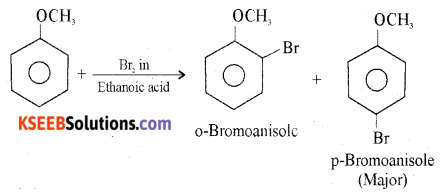Question 34.
(a) How is benzoyl chloride converted into benzaldehyde? Write the equation and name the equation.
When benzoyl chloride (acid chloride) is hydrogenated with catalyst palladium on barium sulphate, benzaldehyde is obtained.(b) Write a general equation for the formation of carboxylic acid from the Grignard reagent.
Grignard reagents react with solid carbon dioxide (dry ice) to form salts of carboxylic acids, which on acidification gives carboxylic acid.(c) Complete the reactionQuestion 35.
(a) Mention the I.U.P.A.C. name of (CH3)2 – N – CH3.
Common name: Trimethylamine
IUPAC Name: N, N-Dimethylmethanamine(b) How is Aniline is prepared from nitrobenzene?
Nitro compounds are reduced to amines by passing hydrogen gas in the presence of finely divided nickel, palladium or platinum catalyst.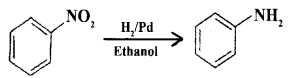(c) Give the equation for the conversion of aniline to 4-Bromo aniline.Question 36.
(a) Write a chemical reaction to ellucidate

1. Glucose contains five -OH groups.
2. Glucose contains six carbon atoms in a straight chain.

1. Acetylation of glucose with acetic anhydride gives glucose Penta acetate which confirms the presence of five OH groups.
2. its molecular formula is found to be C6H12O6

(b) Explain the denaturation of proteins with examples.
When protein is subjected to change in temperature and change in pH, it loses its biological activity. This is called denaturation of protein During denaturation primary structure will not be affected.
Example:

• Coagulation of egg white
• Curdling of milk.

(c) Name the sugar moiety present in DNA.
β-D-2-deoxyriboseQuestion 37.
(a) Name the monomers present in the following polymers.
(i) PVC
(ii) Neoprene
(iii) Nylon-6